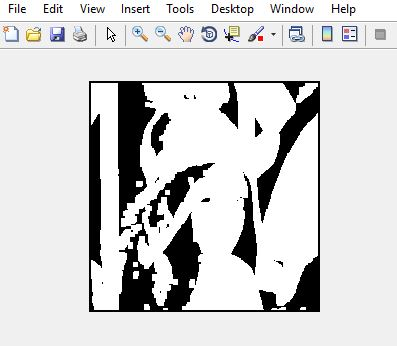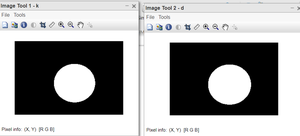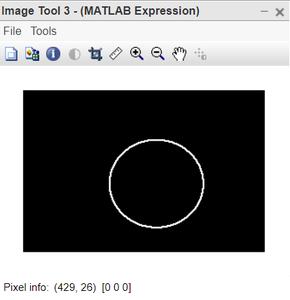# Matlab | Dilation of an Image

• Last Updated : 23 Mar, 2022

Morphology is known as the broad set of image processing operations that process images based on shapes. It is also known as a tool used for extracting image components that are useful in the representation and description of region shape.

The basic morphological operations are:

• Erosion
• Dilation

Dilation:

• Dilation expands the image pixels i.e. it is used for expanding an element A by using structuring element B.
• Dilation adds pixels to object boundaries.
• The value of the output pixel is the maximum value of all the pixels in the neighborhood. A pixel is set to 1 if any of the neighboring pixels have the value 1.

Approach:

• Using function im2bw(), convert the RGB image to a binary image.
• Create a structuring element or you can use any predefined mask eg. special(‘sobel’).
• Store the number of rows and columns in an array and loop through it.
• Create a zero matrix of the size same as the size of our image.
• Leaving the boundary pixels start moving the structuring element on the image and start comparing the pixel with the pixels present in the neighborhood.
• If the value of the neighborhood pixel is 1, then change the value of that pixel to 1.

Example:

## MATLAB

 `% MATLAB code for Dilation``% read image` `I=imread(``'lenna.png'``);    ` `% convert to binary``I=im2bw(I); ` `% create structuring element            ``se=ones(5, 5);` `% store number of rows in P and``% number of columns in Q.          ``[P, Q]=size(se);` `% create a zero matrix of size I.       ``In=zeros(size(I, 1), size(I, 2));` `for` `i=ceil(P/2):size(I, 1)-floor(P/2)``    ``for` `j=ceil(Q/2):size(I, 2)-floor(Q/2)` `        ``% take all the neighbourhoods.``        ``on=I(i-floor(P/2):i+floor(P/2), j-floor(Q/2):j+floor(Q/2)); ``       ` `        ``% take logical se``        ``nh=on(logical(se));   ` `        ``% compare and take minimum value of the neighbor``        ``% and set the pixel value to that minimum value.   ``        ``In(i, j)=max(nh(:));     ``    ``end``end` `imshow(In);`

Output:Figure: Input imageFigure: Output image

Let’s take another example for dilation.

Syntax:

• strel() function is used to define the structuring element. We have chosen a disk-shaped SE, of radius 5.
• imdialate() function is used to perform the dilation operation.
• imtool() function is used to display the image.

Example:

## Matlab

 `%  MATLAB code for Dilation``% read the image.``k=imread(``"dilation.png"``);` `% define the structuring element.``SE=strel(``'disk'``,5);` `% apply the dilation operation.``d=imdilate(k,SE);` `%display all the images.``imtool(k,[]);``imtool(d,[]);` `%see the effective expansion``% in original image``imtool(d-k,[]);`

Output:Figure: Left: Original image, Right: Dilated imageFigure: Expansion in the original image

Code Explanation: